# AP Calculus AB Practice Test 1

### Test Information10 questions20 minutes

Calculator Disallowed

1. If f(x) = 5x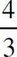, then f ′(8) =

2.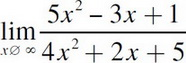is

3. If f(x) =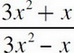, then f′(x) is

4.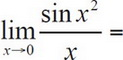5. If x2 - 2xy + 3y2 = 8, then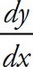=

6.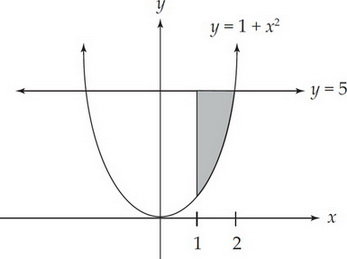Which of the following integrals correctly corresponds to the area of the shaded region in the figure above?

7. If f(x) = sec x + csc x, then f′(x) =

8. An equation of the line normal to the graph of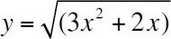at (2, 4) is

9.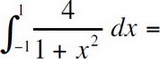10. If f(x) = cos2x, then f′′(π) =